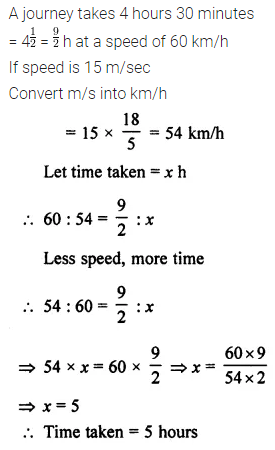ML Aggarwal Class 7 Solutions Chapter 6 Ratio and Proportion Check Your Progress for ICSE Understanding Mathematics acts as the best resource during your learning and helps you score well in your exams.

## ML Aggarwal Class 7 Solutions for ICSE Maths Chapter 6 Ratio and Proportion Check Your Progress

Question 1.
A rectangular park is 120 m long and 75 m wide. Find the ratio of:
(ii) length to its perimeter
Solution: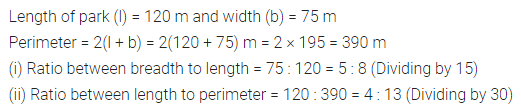Question 2.
Divide the angles of a triangle in the ratio 2 : 3 : 4.
Solution:Question 3.
Heights of Anshul, Ankita and Dhruv are 1.04 m, 1.30 m and 91 cm respectively. Divide 100 sweets among them in the ratio of their heights.
Solution: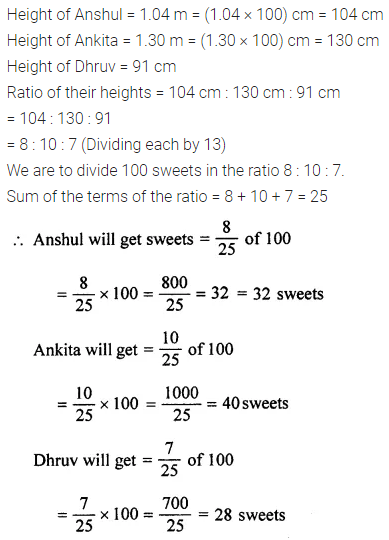Question 4.
The weights of Divya and Himanshu are in the ratio 5 : 7. If Himanshu weighs 28 kg, find the weight of Divya.
Solution:Question 5.
Which ratio is smaller- 9 : 13 or 7 : 11 ?
Solution: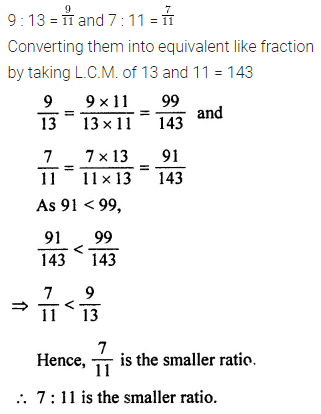Question 6.
Find the fourth proportional to
(i) 4, 7, 20
(ii) 2$$\frac { 1 }{ 2 }$$, 1$$\frac { 1 }{ 4 }$$, 2.2
Solution: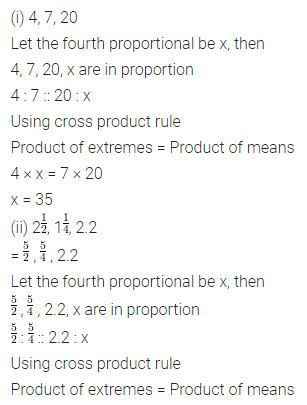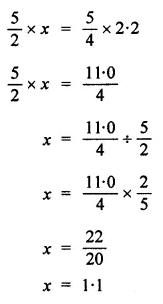Question 7.
A typist types 70 pages in 3 hours 30 minutes. How long will she take to type 300 pages?
Solution: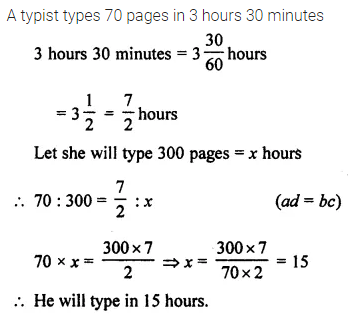Question 8.
12 looms weave 210 m cloth per day. How many metres of cloth will 8 looms weave per day?
Solution:Question 9.
A journey takes 4 hours 30 minutes at a speed of 60 km/h. How long will the same journey take at a speed of 15 m/sec?
Solution: